﻿ Profitability Optimization of Construction Project Using Genetic Algorithm Cash Flow Model

### Profitability Optimization of Construction Project Using Genetic Algorithm Cash Flow Model

Ismail M. Basha, Ahmed H. Ibrahim, Ahmed N. Abd El-Azim

## Profitability Optimization of Construction Project Using Genetic Algorithm Cash Flow Model

Ismail M. Basha1, Ahmed H. Ibrahim1, Ahmed N. Abd El-Azim1,1Construction Engineering &Utilities Department, Zagazig University, Zagazig, Egypt

### Abstract

Cash issues are various and complicated. The contractor starts with a forecast for the flow of the cash through the lifetime of the project. Cash shortages can lead to project failure and business bankruptcy. Researchers have studied cash flow in the context of project delay, business failure, and forecasting. However, negative cash flow trends and patterns themselves are not closely examined despite the amount, duration and distribution of negative cash flow are critical factors in construction performance. This study investigates cash flow management and profit optimization by reducing the extent and amount of negative cash flow on the construction projects and completes the project as scheduled by rescheduling construction activities based on the minimum cash flow availability. The study utilizes genetic algorithm’s technique to devise finance-based schedules that minimize project negative cash flow and profit optimization by identifying the amount and timing of individual inflow or outflow at the end of each period. The study also presents a case study project to illustrate the capability of the proposed model and adopts various constraints, including project profit and due dates, for scenario analysis. The analysis result demonstrates that minimizing negative flow ensures smooth financial pressure by properly shifting activities, and assigning due dates for projects helps planners avoid project duration extension while maximizing overall project profit.

• Ismail M. Basha, Ahmed H. Ibrahim, Ahmed N. Abd El-Azim. Profitability Optimization of Construction Project Using Genetic Algorithm Cash Flow Model. American Journal of Civil Engineering and Architecture. Vol. 4, No. 1, 2016, pp 17-27. http://pubs.sciepub.com/ajcea/4/1/3
• Basha, Ismail M., Ahmed H. Ibrahim, and Ahmed N. Abd El-Azim. "Profitability Optimization of Construction Project Using Genetic Algorithm Cash Flow Model." American Journal of Civil Engineering and Architecture 4.1 (2016): 17-27.
• Basha, I. M. , Ibrahim, A. H. , & El-Azim, A. N. A. (2016). Profitability Optimization of Construction Project Using Genetic Algorithm Cash Flow Model. American Journal of Civil Engineering and Architecture, 4(1), 17-27.
• Basha, Ismail M., Ahmed H. Ibrahim, and Ahmed N. Abd El-Azim. "Profitability Optimization of Construction Project Using Genetic Algorithm Cash Flow Model." American Journal of Civil Engineering and Architecture 4, no. 1 (2016): 17-27.

 Import into BibTeX Import into EndNote Import into RefMan Import into RefWorks

### 1. Introduction

Cash is the most crucial resource of all the project resources, as companies tend to fail due to a deficit in their budget rather than the inadequacy of other resources. Park and Russell stated that over 60% of contractors failures are due to economic factors. There are two main objectives beyond carrying out a financial feasibility analysis: First is to find out if the contractor's available funds are enough to execute the project or not. The second objective is to draw a picture of the extent of utilizing the investment and the progress of payments at different stages of the project. Once these two objectives are obtained, the contractor starts balancing his expenditures throughout the project with his expected available funds, and this is what is known by cash flow management. Ahuja .

Usually the contractor is required to submit an estimate of the progress of payments through the project. This enables the owner to have a solid idea of his own cash requirements. Through the project, the contractor monitors the actual inflow and outflow of the cash, and keeps updates of his predefined estimated cash flow profile.Cash flow and profitability are interactive, even though they are different issues. Wenhua et al. .

The contractor then starts investigating all possible sources of finance to run the project. For him, there are other sources of finance other than his own investment. Such finance comes from banks and financial institutions, which in turns demand an estimate for the contractors needed funds and their timings through the project duration. Once the contractor secured the needed cash, he starts managing the cash profile by trying to meet two goals: first balancing inflow against outflow; second minimizing interest on borrowings.Ahuja .

Due to the importance of project finance, therefore, numerous researchers: Barbosa and Pimentel ; Chiu and Tsai ; Chen et al. ; Park et al. ; Elazouni and Gab-Allah ; Elazouni and Metwally ; Liu and Wang [12, 13] employed various techniques and tried to solve project scheduling problems by integrating scheduling and financial factors to help contractors assessing the overall performance when minimizing the project duration or maximizing project profit. Briefly, the previous investigations has revealed the significance of cash flow in project scheduling and identified critical financial influences, such as credit limit (CL) and payment condition, for practitioners involved in construction.

The previous developed models have limitations such as:

(1) They only consider the extension in the project duration, subject to a certain credit limit. This means that for every credit limit, different completion times are considered in search of the optimum. However, this is not always the case when considering a real-life project. The contractor first needs to know the required funds to complete the project on time. In other words, he wants to know the credit limit that guarantees to complete the project in normal duration. (2) They require double handling of data in exporting the objective function and constraints to optimization software. (3) They need to modify the results of the scheduling software according to the output of the optimization software.

This study aims to solve project scheduling problems by integrating scheduling and financial factors to assist contractors in managing negative cash flow of the project and satisfy the contractor cash limit.

### 2. Cash Flow

Cash flow is a financial model necessary to count the demand for money to meet the project cost and the pattern of income it will generate, Smith . Therefore, the use of cash flow technique is beneficial for the project in both the tender stage and during the project construction progress, where the contractors want to make sure that their planned cash funds is sufficient to cover any possible financial deficit of the project.

### 3. Negative Cash Flow

Cash flow isviewed in two ways in the construction management literature. The first defines cash flow as the net receipt (cash in) or net disbursement (cash out) resulting from receipts and disbursements occurring in the same interest period. According to this school of thought, positive cash flow indicates a net receipt in a particular period or year, whereas negative cash flow indicates a net disbursement in that period. The second construction management view of cash flow defines it as the actual movement or transfer of money into or out of a company. According to this school, money flowing intothe business is termed positive cash flow and is credited as cash received. Money paid out is termed negative cash flow and is debited to the business. The difference between positive and negative cash flows is termed net cash flow. This study adopts the first definition of cash flow because it is accepted by most contractors, widely used in the construction industry, and affirmed by much recent research. Khalil et al .Download asVeiw figureFigures index
Figure 1. Cash flow and Net cash flow profile

Net cash flow is resulted from subtraction of cashout from cashin. Then, the maximum negative cash flow value can be known. This value informs the contractor with the maximum amount of money he needs and its time as shown in Figure 1.

The maximum negative cash flow lies in the 4th interval of time as the maximum amount of money needed along the project life cycle. Hossam Toma .

### 4. Finance-based Scheduling

Establishing bank overdrafts have been one of the prevalent methods of financing construction projects, Ahuja . Finance-based scheduling enables producing schedules that correspond to overdrafts of desired credit limits. Control of the credit limit of an overdraft provides many benefits including negotiating lower interest rates with bankers, setting favorable terms of repayment, and reducing penalties incurred for any unused portions of overdraft cash. In addition, the ability to adjust credit limits helps contractors avoid the phenomenon of progressive cash deficit. This situation occurs when cash available in a given month does not allow the scheduling of much work. During the next month, when a reimbursement is expected, the generated income allows scheduling less work and so forth.

### 5. Cash flow and Project Scheduling

The critical path method/program evaluation and review technique (CPM/PERT) is widely accepted and employed by the construction industry to minimize the total project duration. Elazouni and Gab-Allah  state that many heuristic, optimal, and suboptimal methods have been developed to modify CPM/PERT, yet no oneconsiders cash flow availability as a variable in balancing project expenditures. Using integer programming, they propose finance-based scheduling for devising CPM/PERT so that projects can be adequately financed at certain credit limits.

Genetic algorithms were also used to devise finance-based schedules to maximize project profit through minimization of financing costs and indirect costs. Elazouni and Metwally .

Shuand Chang  used constraint programming to apply finance-based scheduling for multiple projects. In summary, numerous researchers have examined the scheduling problem, but still there is no agreement on the most suitable technique for most construction projects.

### 6. Finance-based Scheduling and Project Profit

As outlined before, credit limits affect project financing costs, indirect costs, and ultimately profit. So, control of the required credit limit enables schedulers to devise schedules that maximize project profit. Finance-based scheduling provides the needed control through devising the minimum-duration project schedule, which corresponds to the credit limit of the minimum interest rate. Thus, finance-based scheduling was used as a tool to minimize project indirect costs, financing costs, and consequently maximize the project profit. Elazouni and Metwally .Download asVeiw figureFigures index
Figure 2. Financial terms of period t considering accumulated financing cost

A cash flow profile is shown in Figure 2 for a project of L periods. The cash flow profile is visualized from the contractors’ perspective. The financial terms that will be used in the model are defined in the following section.

A project total-cost disbursements during a typical project period t, typically, 1 month, is represented by Et which calculated by summation of total direct cost and indirect cost in the same period t. Equation (1) is used to calculate Et.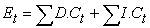(1)

Where: t: one period time of the project, E: total expenses, D.C: direct cost of activities, I.C: indirect cost of the project.

The timing of receiving a payment is at the end of the next period, and the payment amount is represented by Pt which calculated by adding markup to the total expenses of the previous period (t-1) and subtraction the retention value, as shown in equation (2).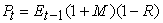(2)

Where: P: payment, E: total expenses of the previous period, M: mark up, R: retention.

However, if the contractor pays the periodical interest on the accumulated negative cash flow (overdraft) O with an interest rate i, the total interest charges at the end of period t is represented by It which calculated by equation (3).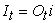(3)

Where: I: total interest charges, O: overdraft, i: interest rate per period t.

Total negative cash at the end of each period t including accumulated interest charges It is represented by Tt which calculated by equation (4).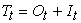(4)

Where: T: total negative cash flow for period t.

Equation (5) calculates the net cash flow (residue) at end of period t after receiving a payment Pt which represented by RSt .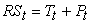(5)

Where: RS: residue.

### 7. Genetic Algorithm (GA)

John Holland invented genetic algorithm (GA) in 1975. GA is a metaheuristic that simulates Darwin's theory of evolution and the survival of the fittest. Evolution as a theory suggested that living things breed and upgrades through reproduction, mutation, and crossover of inherited genes. Kalyanmoy Deb . The metaheuristic adopts randomly guided search to find global optimum values for combinatorial optimization problem. The basic principle of GA is to transform the random search process into non-random ones so that the improvement occurs in the first generation of the solution becomes the basis for the next one.

The first step in solving any combinatorial optimization problem using GA is the formulation and the encoding of the parameters of the problem in the form of strings referred to as "chromosomes". The genes are then created in random matter; this means that the parameters encoded are randomly based, and that every gene presents one possible solution of the problem. In this study, the gene structure is set as a string of elements, each corresponding to a start time assigned to an activity, as shown in Figure 3. As such, each gene represents one possible schedule. To evaluate genes, the evaluation criterion is set as the expected contractor’s profit and negative cash flow at the end of the project.

The objective of the GA proposed in this study is to search for a project schedule, which minimizes the negative cash at any period t and optimizes project profit. Good chromosome individuals are those that yield high values in case of a maximization problem or low ones, if the problem is a minimization. Goldberg .Download asVeiw figureFigures index
Figure 3. Gene formation in this research

The number of chromosomes generated represents the population size. The population size usually affects both the run time and the precision of the solution. It is determined by trial and errors. A whole, complete cycle of creation of chromosomes, their evaluation against the fitness function, and finally the selection of the fittest is referred to as one generation (iteration). The number of generations also affects both the run time and the precision of the solution. Likewise the population size, the appropriate number of generations is determined experimentally.

The first generation of chromosomes yields a number of offspring generations. Only good ones are selected and the rest is discarded. Then the offspring generation undergoes reproduction, crossover, and mutation in an attempt of enhancing the solution. A next generation of offspring is then created and evaluated against the fitness function. This process continues until the termination condition is satisfied.

Reproduction is the process of selecting chromosomes with the highest fitness values and moving them to the next generation. They act as parents for the new offspring generation, and thus contribute in the bitterness of the solution. The chromosomes of lowest fitness are discarded to keep the number of population constant.

Crossover is the process of mixing two chromosomes in an attempt of exchanging their qualities randomly. It is considered the most important operation in genetic algorithm. Crossover mimics marriage, where two parent chromosomes are selected at random and exchange information about the problem at hand. The randomization in selecting chromosomes is guided by the probability of selecting a chromosome; fittest chromosomes are more likely to be selected.

Mutation is the resemblances of sudden evolve of a certain offspring within a generation that turns to be a genius. The logic behind the process of mutation is that the continuous generations of chromosomes might be lacking a piece of information without which achieving optimality is not possible. No matter how many reproduction and crossover processes take place, such piece of information will still be missing. In order to account for the missing piece of info, some chromosomes are then muted. The number of chromosomes to be muted is determined according to the mutation probability Pm, which is usually less than 5% of the total population of chromosomes. Figure 4 illustrates the process of crossover and mutation.Download asVeiw figureFigures index
Figure 4. Crossover process between two chromosomes and mutation process of a chromosome

The offspring chromosomes are compared with the worst value chromosomes and replace them if they give better values; otherwise, they are discarded. The process is repeated until a termination criterion is satisfied. In this research, the termination condition is set to be the number of generation cycles.

### 8. Model Methodology

As outlined before, negative cash flow affects project financing costs and ultimately profit. Therefore, control of the negative cash flow enables schedulers to devise schedules that maximize project profit. This system is a quantitative system design and attempts to minimize negative cash to avoid a budget deficit without delaying project completion, thereby helping contractors release financial pressures on activity execution. This is done by attempting minimizing cash overdrafts in case of borrowing.

Hence, maximum profit can be attained. This necessitates an appropriate cash flow management strategy Figure 5 shows a chart for this system methodology.Download asVeiw figureFigures index
Figure 5. Chart for Model Methodology

The main objective of the problem can be stated as optimizing the project profit by:

(1) The project is initially scheduled according to input data, such as activities’ relations and durations

(2) Cash flow is forecasted according to the initial schedule of the project and maximum negative cash flow and profit is determined.

(3) Optimization process using GA starts to search for the best scenario of the project according to predefined objective function. In this step, Cash flow is forecasted with activities available floats are utilized to generate multiples of scenarios with activities starting at different times. Project scenarios are generated using GA processes which are reproduction, crossover, and mutation as discussed before. Then each scenario is evaluated against a predefined objective function in a search of the optimum start time values according to this objective function. The methodology adopted in accomplishing this goal is to integrate various cash problem in one complete model. To do this, three scenarios are adopted. The first scenario is used to search for a solution for the problem of devising schedules that correspond to minimum negative cash flow without reducing the profit. The second scenario is used to search for a solution for the problem of devising schedules that correspond to a specified predefined negative cash flow. The last scenario is used to search for a solution for the problem of devising schedules that correspond to the specified predefined profit.

(4) Model output data are optimized schedule, optimized cash flow, and net cash flow diagram for the selected scenario.

To illustrate the model methodology a practical construction projectis tested to ensure the capability of the proposed model in the next section.

### 9. Project Case Study

This study uses a sample project to illustrate the capability of the proposed model and adopts various constraints, including project profit and due dates, for scenario analysis. The project is the maintenance and restoration of an old primary school (Omar El-Farouq primary school-Ismailia City). This project belongs to the general authority of educational buildings; the school building consists of two stores with area 4000m2.

The project is assigned to one main contractor and includes the following works: Removal of damaged items, installation of new items and maintain some damaged items.

Table 1 shows the items of works, duration and direct cost of each item and Figure 6 shows the planned activity network of the project.

Application of the method involved three steps: Initial schedule; forecasting initial cash flow; optimizing cash flow according to various constraints. The methodology adopted in accomplishing this goal is to integrate various cash problem in one complete model. To do this, three scenarios are adopted. The first: Minimizing negative cash flow with optimum profit, the second: Set a specified negative cash flow limit and the third scenario: Set a specified Profit limit.

#### Table 1. Project items of works and other essential dataDownload asVeiw figureFigures index
Figure 6. Project case study network
9.1. Initial schedule

The data required to run the interface program are shown in Figure 7, which represents an input screen of four columns of input data. First column, ‘‘Activity Name,’’ is used to identify the activities of the project; Second column, ‘‘Duration,’’ is the activity duration in working days or months; Third column “Predecessor,” is used to define the precedence relationships between activities; Fourth column “Direct Cost,” is the direct cost per unit time of each activity.

Figure 8 shows the initial schedule of theproject, which is basically a bar chart with a working day as the time unit with total floats portrayingafter activities. The scheduling software’s initial solution represented the early-start schedule.

(Appendix Table A1) shows time data of the initial schedule according to data which inserted in the input screen in Figure 7 such as EST=early start time, EFT=early finish time, LST= late start time, LFT= late finish time, TF=total float, FF=free float. Note that total float of each activity (TF) is the time which we depend on to shift the activity without project delay.Download asVeiw figureFigures index
Figure 7. Essential Project schedule Data (Input data)Download asVeiw figureFigures index
Figure 8. Initial schedule of example CPM method (Output data)

#### Table 2. Cash flow calculation according to initial schedule (Output data)

9.2. Forecasting Initial Cash Flow

Figure 9 shows input screen to insert the payment terms of the contract and cost-estimating information such as indirect cost, markup, initial payments, retention, interest rate on debit.Download asVeiw figureFigures index
Figure 9. Financial data for cash flow (Input data)

Table 2 shows cash-in and cash-out values and values of the other financial parameters along the duration of the project considering borrowing interest rate of 1% per month. The calculation of the maximum negative cash flow 30508.28 at the end of 14th month and project profit 2797.837. The output screen of the net cash flow profile according to previous calculation in Table 2 is shown in (Appendix Figure B1). Note: The cash flow calculations in Table 2 are calculated according to the initial schedule before GA optimization. Calculations show that: Maximum negative cash flow 30508.28 at the end of 14th month and project profit 2797.837. The GA system can then be used to search for optimum schedules that minimize maximum negative cash flow and optimized project profit.

Explanation of Table 2 calculations: residue amount (bolted cell as a reference) has been calculated according to the bank loan transactions as follows:

1. An overdraft has been made from the previous month (9,000) and an interest has been added to the overdraft (90), that concludes Total bank's Debit of 9,090.

2. Overdraft of the current month calculated by adding the residue amount from the previous month to the new expenses of the current month (9,090+9,000).

3. An interest has been added to the new total overdraft (18,090+180.9).

4. The residue amount for the current month will concludes by subtracting total cell from the payment has been made to the bank (18,270.9-8,505). …..etc.

9.3. Model Verification

Verification is the process of determining that a model implementation accurately represents the developer’s conceptual description of the model and the solution to the model. The verification of this model is performed by developing a cash flow sheet by Excel 2007 and comparing corresponding results for the same project case study used by the proposed model. The results of using this model are obtained for the same values of all parameters such as the borrowing interest rate, indirect cost, retention and markup which amounted to the same values used in the spreadsheet. It is noted that the results of the proposed model are the same results of sheet, which reflect the ability of the proposed model to predict an accurate project cash flow.

9.4. Optimization Process

The main objective of this study is to develop a tool for optimizing the contractor’s profit. The methodology adopted in accomplishing this goal is to integrate various cash problem in one complete model. To do this, three scenarios are adopted. For implementation purpose, the detailed GA procedure is completely coded using C-sharp and then uses to search for an optimum schedule for the problem at hand.

9.4.1. Scenario I: (Minimizing Negative Cash Flow with Optimum Profit)

For optimizing negative cash flow, a GA technique is used to search for a solution for the problem of devising schedules that correspond to minimum negative cash flow.

The objective and user defined constraints in this scenario are as follows: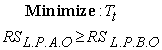Where: RSLPAO: residue in last period after optimization, RSLPBO: residue in last period before optimization, Tt = Negative cash flow for each period t, RSLP: residue in last period= Profit.

Schedules are generated using random start times of activities while maintaining dependency between activities. The corresponding profiles of cash requirements of these randomly generated schedules are produced. Then, the GA procedure searches for the schedule that produces minimum debit value and optimum project profit.

Another input screen shown in Figure 10 prompts user input regarding GA parameters of population size and number of generations. Figure 11 shows the optimized schedule of the project with activities new start dates resulted from optimization engine using GA.

The output screen of optimized net cash flow profile for scenario (I) is shown in (Appendix FigureB2).The model will present cash-in and cash-out values and values of the other financial parameters along the duration of the project considering borrowing interest rate of 1% per month in this scenario.Download asVeiw figureFigures index
Figure 10. Input screen of coded genetic algorithm procedure

9.4.2. Scenario II: (Set a Specified Negative Cash Flow Limit)

In this scenario, a GA technique is used to search for a solution for the problem of devising schedules that correspond to the specified negative cash flow, which has been inserted in the input screen shown in Figure 12 with no constraint on the profit. The user defined constraints in this scenario are as follows: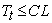Where: Tt= Negative cash flow for each period t, CL: credit limit (Max negative cash entered by user in Figure 12).Download asVeiw figureFigures index
Figure 11. Optimized project schedule for scenario I (output data)Download asVeiw figureFigures index
Figure 12. Input screen of coded genetic algorithm procedure and specified negative cash flow

Figure 13 shows the optimized schedule of the project with activities new start dates resulted from optimization engine using GA for scenario(II).

The output screen of optimized net cash flow profile for scenario II is shown in (Appendix Figure B3). It can be seen from the results in (Appendix FigureB3) that all values of cumulative negative cash fall below the specified credit limit which inserted by the user.Download asVeiw figureFigures index
Figure 13. Optimized project schedule for scenario(II)

9.4.3. Scenario III: (Set a Specified Profit Limit)

In this scenario a GA technique is used to search for a solution for the problem of devising schedules that correspond to the specified profit which inserted in the input screen shown in the Figure 14 without any constraint on the negative cash flow. The profit of the project should not be less than this profit limit.Download asVeiw figureFigures index
Figure 14. Input screen of coded genetic algorithm procedure and specified ProfitDownload asVeiw figureFigures index
Figure 15 . Optimized project schedule for scenario III (output data)

Figure 15 shows the optimized schedule of the project with activities new start dates resulted from optimization engine using GA for scenario(III). The user defined constraints in this scenario are as follows: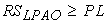Where: RSLPAO: residue in the last period after optimization (Profit), PL: profit limit entered by user in Figure 14.

The output screen of optimized net cash flow profile for scenario III is shown in(Appendix Figure B4).

Table 3 shows the start time of each activity in each optimization scenario. The first scenario is to minimize negative cash flow and keep or maximize the profit, the results shows that negative cash flow minimized from 30508.28 to 25628.76 and the profit maximized from 2797.837 to 2910.475 and the optimized schedule of the project shown in Table 3 shows that activity A, C, D, E, H, I and J start times have changed to reach the optimum schedule with the minimum negative cash flow and optimum profit.

#### Table 3. Compression between initial schedule and optimized schedule for the three scenarios

The second scenario is to reach a specific negative cash with optimum profit in this scenario negative cash flow constraint was not to exceed 27000 and the results shows that maximum negative cash minimized from 2797.837 to 26433.74 to satisfy this constraint and the profit increased to 2869.955, activity F, H and I start times have changed to reach the required negative cash flow.

The last scenario is to reach a specific profit with the optimum negative cash flow this scenario the constraint was on the profit to not be less than 30000 the results shows that the profit increase from 2797.837 to 3001.064 and activities A, C, D, E, H, I and J start times have changed to reach the optimum schedule with the required profit.

### 10. Conclusion

This study considers cash flow, establishes a novel profit optimization model using GA for Project scheduling problems, and performs periodic financial inspection on behalf of the contractor. This work creates an overall time framework and integrates cash flow and financial elements into the model to assist evaluating project financing. Scenario analysis employs an example project for model illustration, and the optimized schedule is conducted to pursue negative cash flow minimization and profit optimization. Possible practical constraints, including due date and initial negative cash and profit are also assigned to the scenario for minimizing negative cash flow, the model capability is demonstrated smoothing financial pressure by shifting activity schedules without delaying completion time. Consequently, the proposed model identifies an appropriate scheduling plan to fulfill contractor financial needs related to schedule problems. The model is verified by comparing its results to the results of Excel sheet and its results are stable. It provides contractors with an invaluable tool for negotiating and establishing good bank overdrafts.

### Declaration

This paper is based on master thesis prepared by the third author (corresponding author) and under supervision of the first two authors.

### Notation

The following symbols are used in this study:

t: one period time of the project.

E : total expenses.

D.C : direct cost of activities.

I.C : indirect cost of the project.

P: payment.

M: mark up.

R : retention.

I: interest.

O : overdraft.

i: interest rate.

T : total negative cash flow for period t.

RS : residue.

RSLPAO : residue in last period after optimization.

RSLPBO : residue in last period before optimization.

Tt: negative cash flow for each period t.

RSLP : residue in last period (Profit).

CL: credit limit.

PL: profit limit.

### References

  Ahuja, H. N. 1983. Project management: techniques in planning and controlling construction projects. John Wiley and Sons, USA.In article  Barbosa, P. S. F., and Pimentel, P. R. 2001. A linear programming model for cash flow management in the Brazilian construction industry. Construction Management And Economics, 19(5): 469-479.In article View Article  Chiu, H. N., and Tsai, D. M. 2002. An efficient search procedure for the resource-constrained multi-project scheduling problem with discounted cash flows. Construction Management And Economics, 20(1): 55-66.In article View Article  Chen, H. L., O’Brien, W. J., and Herbsman, Z. J. 2005. Assessing the accuracy of cash flow models: The significance of payment conditions. Journal of Construction Engineering and Management, 131(6): 669-676.In article View Article  Elazouni, M. A., and Gab-Allah A. A. 2004. Finance-based scheduling of construction project using integer programming. Journal of Construction Engineering and Management, 130(1): 15-24.In article View Article  Elazouni, M. A., and Metwally, F. G. 2005. Finance-based scheduling: tool to maximize project profit using improved genetic algorithms. Journal of Construction Engineering and Management, 131(4): 400-412.In article View Article  Goldberg, D.E. 1989. Genetic algorithms in search, optimization, and machine learning. Addison-Wesely Publishing Company, Inc., New York.In article  Park, H.K.and Russell, J.S. (2005).Cash flow forecasting model using moving weights of cost categories for general contractors on jobsite. First International Conference in the 21s' Century, Florida International University, Miami, Florida.In article  Hossam, M. W. 1997. Development of a computer model for prediction of construction contracts cash flow. Master Of Science Structural Engineering, Faculty Of Engineering , Cairo University, Giza, Egypt.In article  Kalyanmoy, D. 2002. Genetic algorithm in search and optimization: the technique and application. India Institute of Technology, Kanpur, Up208 026, India.In article  Khalil, A., Raid, A., and Mohammed E. 2012. Analyzing the impact of negative cash flow on construction performance in Dubai area. Journal of Construction Engineering and Management, 382-390.In article  Liu, S. S., and Wang, C. J. 2008. Resource-constrained construction project scheduling model for profit maximization considering cash flow. Autom. Constr., 17(8), 966-974.In article View Article  Liu, S. S., and Wang, C. J. 2009. Two-stage profit optimization model for linear scheduling problems considering cash flow. Construction Management And Economics, 27(11), 1023-1037.In article View Article  Shu, S. L., and Chang, J. W. 2010. Profit optimization for multiproject scheduling problems considering cash flow. Journal of Construction Engineering and Management, 136(12), 1268-1278.In article View Article  Smith, N. J., 2008. Engineering project management. Third Edition, Blackwell Publishing.In article  Wenhua, H., Xing L., and Deqiang C. 2011. Payment problems, cash flow and profitability of construction project: a system dynamics model. World Academy of Science, Engineering and Technology 58: 693-699.In article

### Appendix BDownload asVeiw figureFigures index
Figure B1. Initial net cash flow (output data)Download asVeiw figureFigures index
Figure B2. Optimized project cash flow chart in scenario I (output data)Download asVeiw figureFigures index
Figure B3. Optimized project cash flow chart for scenario II (output data).Download asVeiw figureFigures index
Figure B4. Optimized project cash flow chart in scenario III (outputdata)